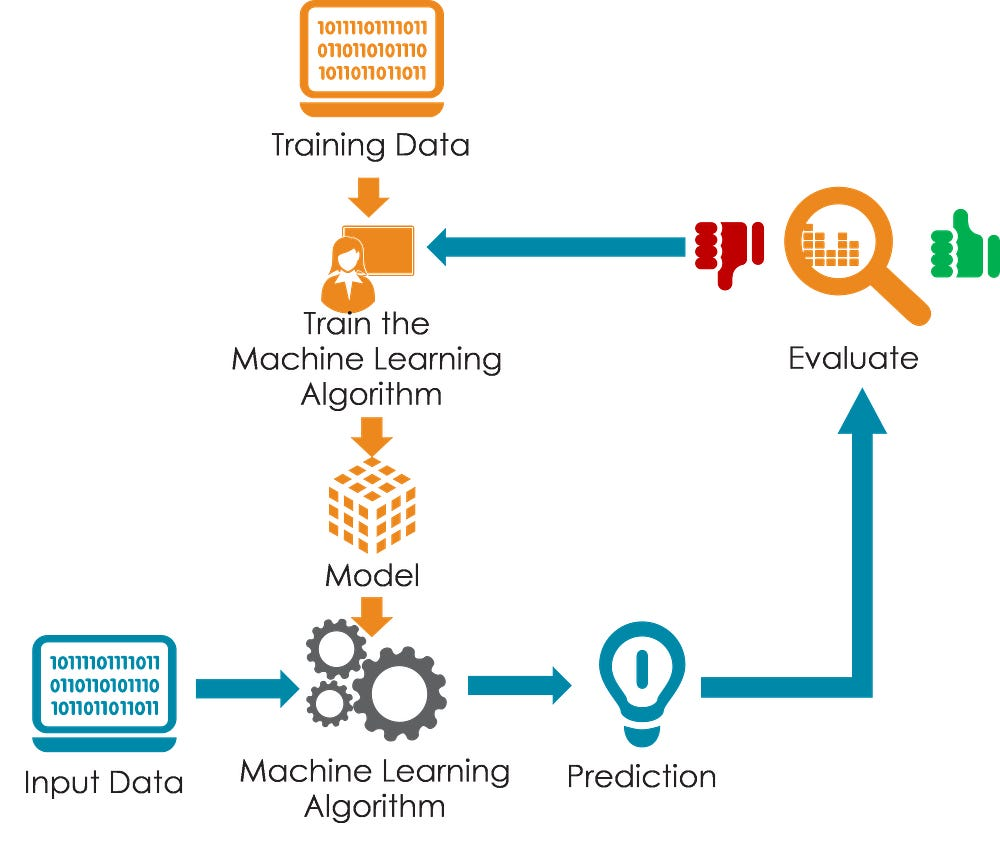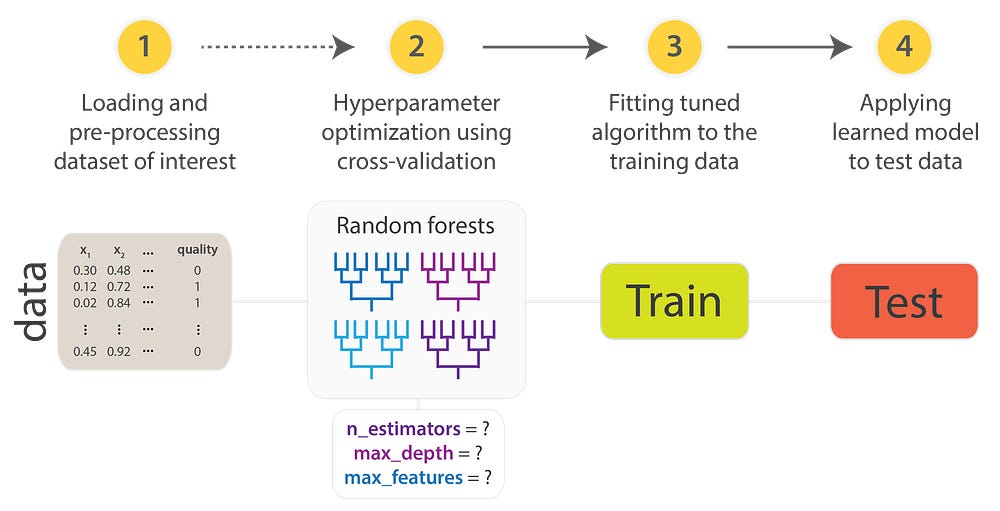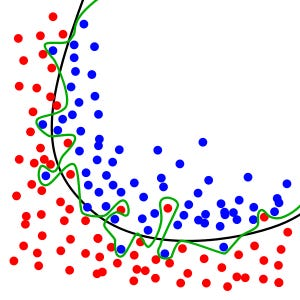# Train, Validation, Test Set in Machine Learning— How to understand

Source: Deep Learning on Medium

Train, Validation, and Test Set are three of the biggest jargons in Machine Learning and AI. Seemingly, many misunderstand it. When I ask some of my friends about the differences between train, validation and test set, they can’t answer it. Today I will explain you all about three above terminologies.

Training Set

You can imagine that machine learning algorithm as a student in class and data is the knowledge given by the teacher. The teacher uses knowledge to teach student solving a problem. In machine learning, the training set is known from the teacher to teach the student. Student (machine learning model) try to remember and find out insights from training set then store those insights to its parameters (or weight) by using optimization algorithms. The ability of student reflects through the training error. The student has a lower training error is better than one has higher training error. However, remember that our final goal is to find the student that works well in unseen data, I mean the data in the future.

Validation Set

Validation set, sometimes called Development set (Dev set). The main purposes of dev set are preventing the machine from overfitting and choosing hyperparameters. Preventing machine from overfitting helps machine learning model work better with future data as well as preventing the student from learning by rote. Choosing hyperparameters helps to find the best machine learning algorithm of the rest as well as find the best student in the class who have a specific gift.
Choosing hyperparameters: beside parameters learned from train data, each machine learning algorithm usually has some hyperparameters. That hyperparameters must choose manually. In the real world, we have many types of data from marketing place to NLP, medical and so on. Each type of data as a subject in school. And each student is good at a specific subject because of his hobby and his gift. So that teacher in class must use some dev set to find the best student for each type of subject.Illustration for choosing hyperparameters for machine learning algorithm

Prevent overfitting: sometimes, students learn by rote. So that we must to using dev set to test students. Dev set can consider as a test teacher make independently from the train set. As the picture below, dots are training data and curves are your algorithms. The green curve is overfitting and the black is good.Illustration for overfitting problem

Test Set

This dataset is an independent form train and dev set but three datasets must have the same distribution. Imagining, after the student learns from train set and after teacher chooses the best student using dev set. The test set as the exam to check the real ability of student after learning.

Summary

Train set: Using to train and optimize the parameters of the model
Dev set: choose hyperparameters and prevent overfitting
Test set: give the unbiased evaluation for your model

Reference